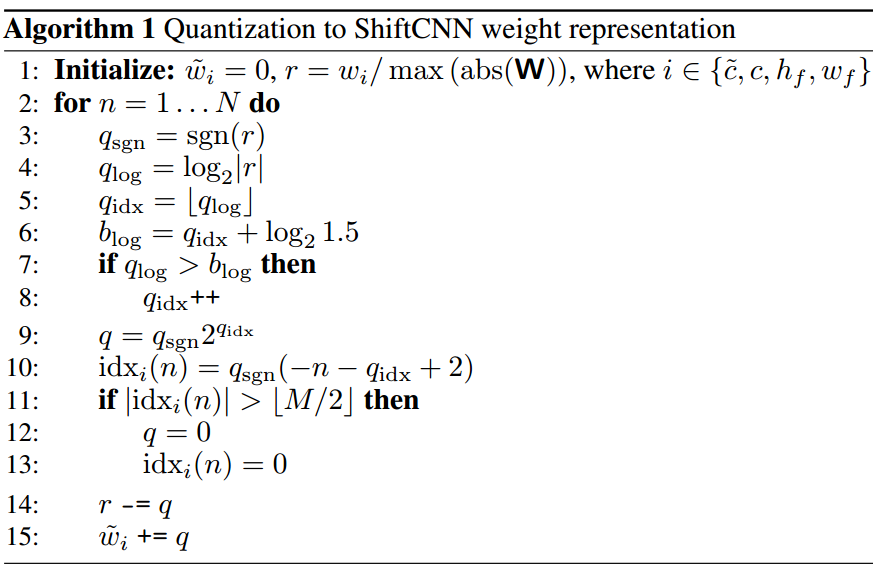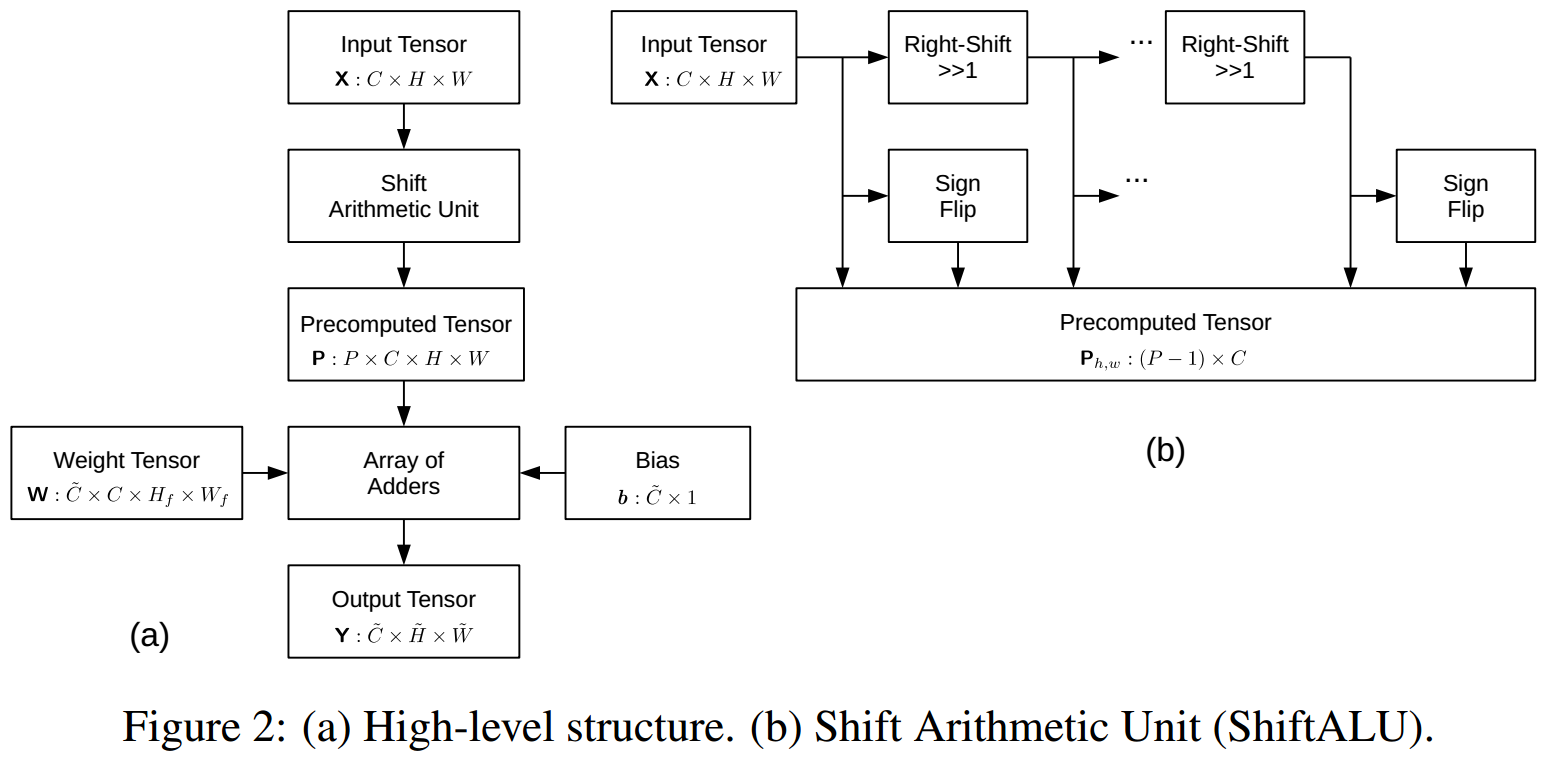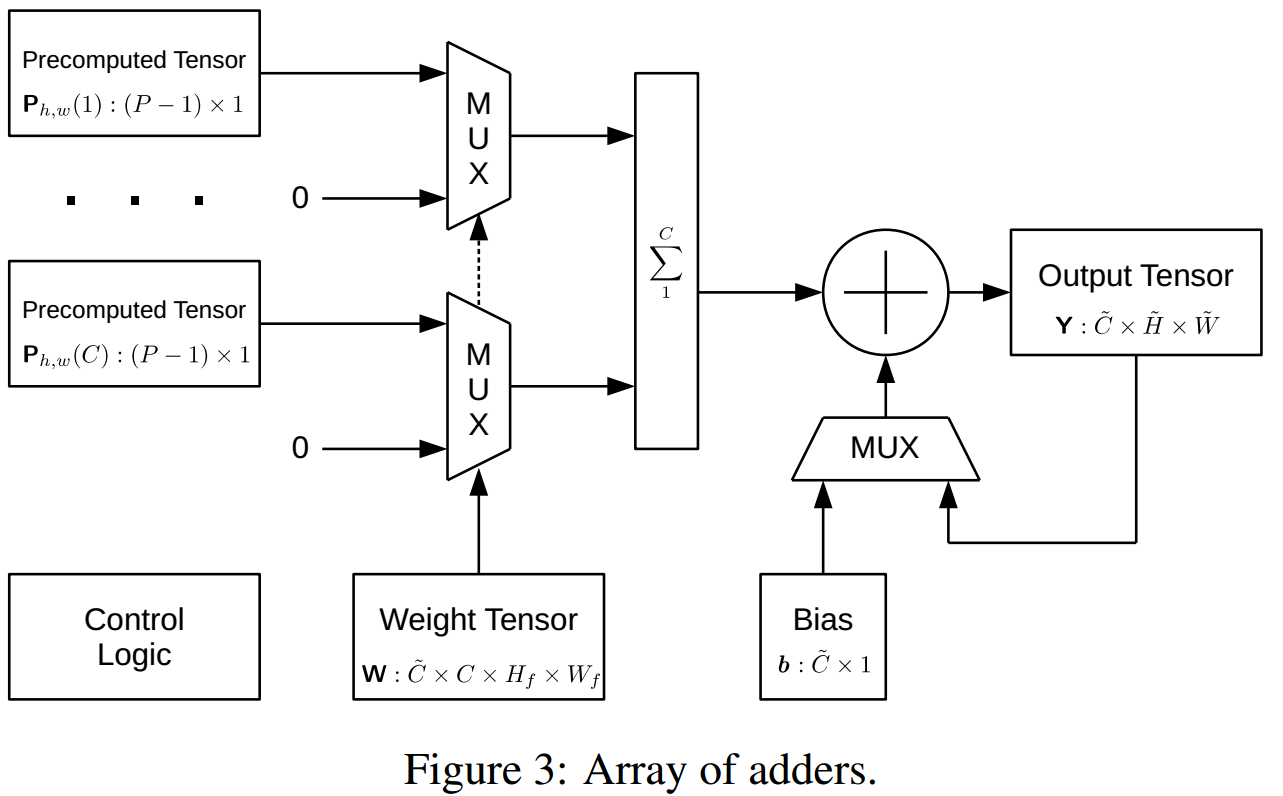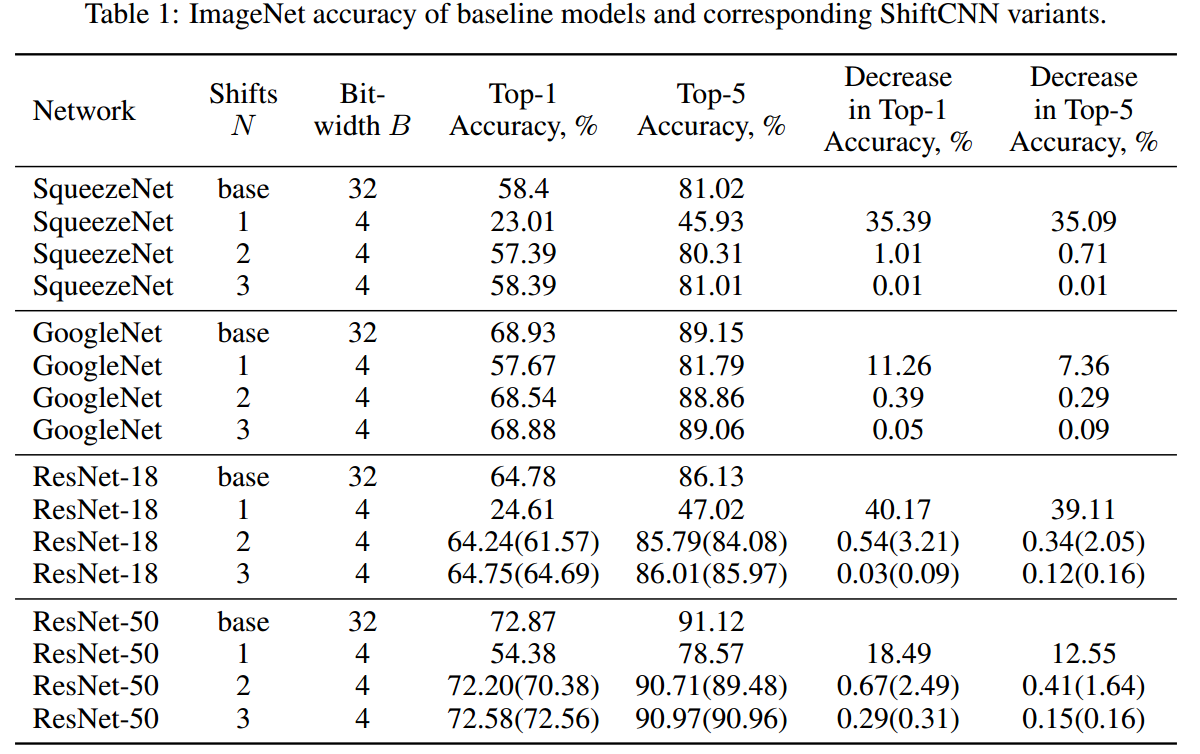# ShiftCNN —— 基于量化的低精度网络表达

(1) 低精度数据表达

(2) 卷积计算方式

(3) 最优量化

### 量化

ShiftCNN所采用是一种相当巧妙的类似于残差量化的方法。

$$C_n = {0, \pm2^{-n+1},\pm2^{-n},…,\pm2^{-n-\lfloor M/2 \rfloor + 2}}$$

$$C_1 = {0,\pm2^{-1},\pm2^{-2},\pm2^{-3},\pm2^{-4},\pm2^{-5},\pm2^{-6}}$$

$$C_2 ={0,\pm2^{-2},\pm2^{-3},\pm2^{-4},\pm2^{-5},\pm2^{-6}},\pm2^{-7}$$

$$\hat{w_i}=\sum_{n=1}^{N}C_n[idx_i(n)]$$### 卷积计算### 实验

ShiftCNN量化后无需再训练。 个人觉得再训练应该会更好一点。©️2019 CSDN 皮肤主题: 编程工作室 设计师: CSDN官方博客